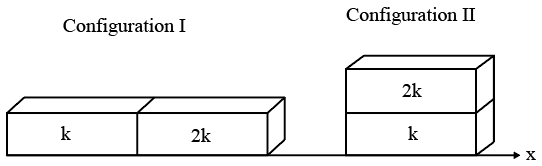Instructions

For the following questions answer them individually

Question 1

# The diameter of a cylinder is measured using a Vernier callipers with no zero error.It is found that the zero of the Vernier scale lies between 5.10 cm and 5.15 cm of the main scale. The Vernier scale has 50 divisions equivalent to 2.45 cm. The $$24^{th}$$ division of the Vernier scale exactly coincides with one of the main scale divisions. The diameter of the cylinderisQuestion 2

# A ray oflight travelling in the direction $$\frac{1}{2} \left(\widehat{i} + \sqrt 3 \widehat{j}\right)$$ is incident on a plane mirror. After reflection, it travels along the direction $$\frac{1}{2} \left(\widehat{i} - \sqrt 3 \widehat{j}\right)$$. The angle of incidence isQuestion 3

# In the Young’s double slit experiment using a monochromatic light of wavelength $$\lambda$$, the path difference (in terms of an integer n) corresponding to any point having half the peak intensity isQuestion 4

# Two non-reactive monoatomic ideal gases have their atomic masses in the ratio 2 : 3. The ratio of their partial pressures, when enclosed in a vessel kept at a constant temperature, is 4 : 3. The ratio of their densities isQuestion 5

# Two rectangular blocks, having identical dimensions, can be arranged either in configuration I or in configuration II as shown in the figure. One of the blocks has thermal conductivity K and the other 2K. The temperature difference between the ends along the x-axis is the same in both the configurations. It takes 9 s to transport a certain amount of heat from the hot end to the cold end in the configuration I. The time to transport the same amount of heat in the configuration II isQuestion 6

# A pulse of light of duration 100 ns is absorbed completely by a small object initially at rest. Power of the pulse is 30 mW and the speedoflight is $$3 \times 10^8 ms^{-1}$$. The final momentum of the object isQuestion 7

# A particle of mass m is projected from the ground with an initial speed $$u_0$$, at an angle $$\alpha$$ with the horizontal. At the highest point of its trajectory, it makes a completely inelastic collision with another identical particle, which was thrown vertically upward from the ground with the same initial speed $$u_0.$$ The angle that the composite system makes with the horizontal immediately after the collision isQuestion 8

# The work done on a particle of mass m by a force, $$K \left[\frac {x}{\left(x^2 + y^2\right)^{\frac{3}{2}}}\widehat{i} + \frac {y}{\left(x^2 + y^2\right)^{\frac{3}{2}}}\widehat{j} \right]$$ (K being a constant of appropriate dimensions), when the particle is taken from the point (a, 0) to the point (0, a) along a circular path of radius a about the origin in the x-y plane isQuestion 9

# One end of a horizontal thick copper wire of length 2L and radius 2R is welded to an end of another horizontal thin copper wire of length L and radius R. When the arrangement is stretched by applying forces at two ends,the ratio of the elongation in the thin wire to that in the thick wire isQuestion 10

# The image of an object, formed by a plano-convex lens at a distance of 8 m behind the lens, is real and is one-third the size of the object. The wavelength oflight inside the lens is $$\frac{2}{3}$$ times the wavelength in free space. The radius of the curved surface of the lens isOR